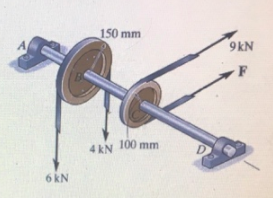# Problem: What is the magnitude of F for the system to remain in equilibrium?a. 5 kNb. 7 kNc. 1 kNd. 6 kN

###### FREE Expert Solution

At equilibrium:

$\overline{){\mathbf{\sum }}{\mathbf{\tau }}{\mathbf{=}}{\mathbf{0}}}$, where Στ is total torque.

Torque is given by:

$\overline{){\mathbit{\tau }}{\mathbf{=}}{\mathbit{r}}{\mathbit{F}}}$, where r is radius and F is force.

82% (272 ratings)###### Problem Details

What is the magnitude of F for the system to remain in equilibrium?a. 5 kN

b. 7 kN
c. 1 kN
d. 6 kN